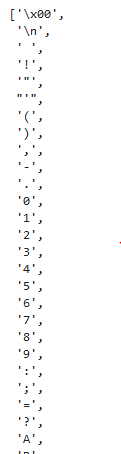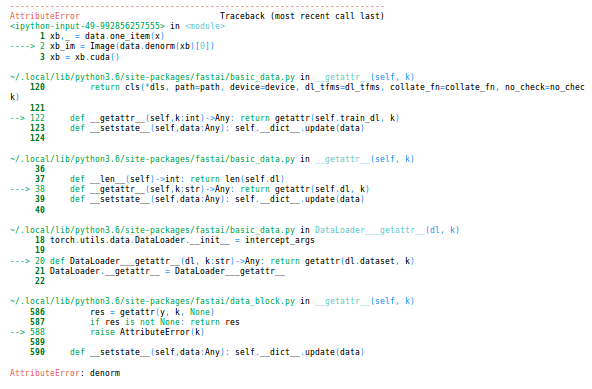# Lesson 6 In-Class Discussion

I really didn’t get the `EmbeddingDotBias` object from this lesson and the “Movie bias” part of the lesson5-movielens notebook.
How is a bias embedding matrix able to tell us what is the best or the worse movie of all times? How can we infer bias = best/worse movie in our case? (btw we did a lookup on the top 3000 movies with `movie_bias = to_np(m.ib(V(topMovieIdx)))` so how are we supposed to find the worse movies?)

It’s even more confusing as @jeremy takes a different approach for “Embedding interpretation” where he goes from plotting the scores of reduced dim of the embedding and then guessing what the relationship between the top and bottom items.

The way I find easiest to understand this is with the spreadsheet where I showed how the dot product and bias fit together. Does anyone else have other ways to think about this which might provide more intuition for @Ekami?

1 Like

Regarding dim=-1 in fastai/courses/dl1/lesson6-rnn.ipynb

Has anybody else run into this error?:
`TypeError: log_softmax() got an unexpected keyword argument 'dim'`

It happens in the last line in the rnn models where the softmax is called:

``````    def forward(self, *cs):
bs = cs.size(0)
h = V(torch.zeros(bs, n_hidden).cuda())
for c in cs:
inp = F.relu(self.l_in(self.e(c)))
h = F.tanh(self.l_hidden(h+inp))

return F.log_softmax(self.l_out(h), dim=-1) <===
``````

Looks like it should be changed to:

``````        return F.log_softmax(self.l_out(h)) <===
``````

it seems that this parameter has been changed in pytorch and should be removed from CharLoopModel, CharLoopConcatModel and CharRnn. It seems that probably the most up-to-date notebook is not in github (?). For now in my local copy I have just removed it. @jeremy do you have a more up-to-date version from the lesson that you could check in?

Thanks

Pytorch 0.3.0’s softmax function accepts the `dim` argument.

You probably need to do a git pull + conda env update. There was an update that switches the pytorch channel from “sousmith” to “pytorch” which results in grabbing pytorch 0.3.0 rather than 0.2.0.

2 Likes

Strange I had updated both fastai and my environment. I saw this post:

so I ran:

``````git pull
source activate fastai
conda env update
``````

and saw that 0.3.0 was installed:
`pytorch-0.3.0- 100% |################################|`

If it is supposed to accept the parameter, that is helpful. I had thought it had been removed. I’ll go through the steps to update again to see if I can get the right behavior. Thanks.

2 Likes

@Ekami, just in case this is still unsolved in your head, after step number one that @jeremy suggested, understanding the spreadsheet, more ideas:

The question is that with “latent factors” of your embedding you are capturing interations between user-movie, that is, how much dialogue does the movie has? interacts with how much does this user love/hate long dialogs? But bias terms are not interacting, they are user specific or movie specific. So, by having a look at the movie biases that your SGD has learnt you are seeing the especific goodness/badness of a movie… more or less.

I say more or less cause the way I see it, more than “the best/worst movies of all times” maybe would be “the best/worst movies in its class”. Or the movies that sharing similar latent factors with others somehow got much better or much worse critics than those other smilar movies. Its a more subtle way of assesing the goodnes of a movie, beyond how many stars it was rated (otherwise you would just take the movies with 1 star as worse and the movies with 5 stars as best with no machine learning at all)

And about reducing dimensionality for representation… I dont think its conceptually different from taking just size two or size three embeddings and watching 2D or ·3D plots to gain intuition about where movies are, not really that confusing4 Likes

Thanks a lot for taking the time to explain it to me @miguel_perez. It’s clearer now but I still feel like I’m missing some pieces such as “what is a latent factor” and few other details. I’ll find them by myself and come back to your explanation. That really helps, thanks a lot1 Like

A factor is something that causes another thing. For example, sunlight causes energy.

A latent factor is also a factor, but it’s hard to measure directly. You know it is present and causing something, but it’s hard to measure it.

In the movies example, “dialogue rich” or “action-comedy” are for example, 2 latent factors for movies. There is no scale to measure them directly other than a real number between 0&1 showing how much of ‘dialogue richness’ or ‘action-comedyness’ is there in the movie.

For users, say 2 latent factors are: ‘love for dialogues’ and ‘aversion for action-comedy type fights’ etc. When you multiply the user factors with the ‘corresponding’ movie factors, and sum them up you get a score which can be equated to a rating that user would give a particular movie.

In collaborative filtering, we go from known ratings to inferring latent factors. Then, using those inferred latent factors, predict the unknown ratings(for recommending movies to users).

Hope this helps.

5 Likes

Thanks a lot for these very clear informations1 Like

I am reviewing RNNs and a have a very basic question. I didn’t quite understand why we are not including the final sequence when creating c_in_dat:

Thanks !

IIRC it’s because the final sequence doesn’t have a label.

I posted this question on Wiki: Lesson 6 topic, but wasn’t able to figure it out. Would anybody point me to the right direction?

Thank you!!

In simplest terms my understanding is that:
Autoencoder reconstructs the input image accurately.
Variational Autoencoder reconstructs variational versions of input image as output, for a given input image there can be multiple images which are close to the input image but with certain difference. In VAE latent space takes up probability distribution.

So when we do this, to_np(m.ib(V(topMovieIdx).cpu())) does this make a prediction for any set of movies?

Hi Friends , I’ve got a question , I’m working on CharSeqRnn. The code is as shown below , the non-overlapping sequence one:-

``````class CharSeqRnn(nn.Module):
def __init__(self, vocab_size, n_fac):
super().__init__()
self.e = nn.Embedding(vocab_size, n_fac)

self.rnn = nn.RNN(n_fac, n_hidden)

self.l_out = nn.Linear(n_hidden, vocab_size)

def forward(self, *cs):
bs = cs.size(0)
h = V(torch.zeros(1, bs, n_hidden))
inp = self.e(torch.stack(cs))
#         print("Input size",inp.size())
outp,h = self.rnn(inp, h)
#         print("Output size",self.l_out(outp[-1]).size())
return F.log_softmax(self.l_out(outp), dim=-1)
``````
``````m = CharSeqRnn(vocab_size, n_fac).cuda()

it = iter(md.trn_dl)
*xst,yt = next(it)
t = m(*V(xst)); t.size()
``````

Then the custom loss function:-

``````def nll_loss_seq(inp, targ):
sl,bs,nh = inp.size()
targ = targ.transpose(0,1).contiguous().view(-1)
return F.nll_loss(inp.view(-1,nh), targ)

fit(m, md, 4, opt, nll_loss_seq)

``````
``````def get_next(inp):
idxs = T(np.array([char_indices[c] for c in inp]))
p = m(*VV(idxs))
i = np.argmax(to_np(p))
print(i)
return chars
``````

When I’m using :-

``````(get_next('for thos'))
``````

When I do `get_next('for thos')`, Its showing all the 85 characters as output. How to get the next predicted character. I tried playing with the dimensions , but couldn’t get the desired next predicted output. . Any help is really appreciated.
The output that I get is shown in the snapshot below:-Thanks,
Ashis

Hi,
I’m trying to create Heatmap based on medical images dataset (when classifying between two tumors types)
running the following (based on lesson6-pets-more notebook)
xb,_ = data.one_item(x)
xb_im = Image(data.denorm(xb))
xb = xb.cuda()

I get denorm related error:Any idea what went wrong?
Thanks a lot
Moran

getting an error on the rossmann data cleaning notebook. It says requirement already satisfied: isoweek.

Then immediately below it says: ModuleNotFoundError: No module named ‘isoweek’

I’ve tried restarting the kernel pip installing isoweek in the terminal, etc. Not sure what’s wrong here.

Any ideas?

Hi Nick, I get the same error - did you ever solve it?

Hey after watching lesson 6 I’m trying to understand how the batchnorm compares to sigmoid function that was described in lecture 4. The sigmoid function mapped those values from -1 to 1 onto 0-5.5 range. However it seems in this lecture the batchnorm does the same thing?

I also would like to ask about CNN. In this recommended article https://brohrer.github.io/how_convolutional_neural_networks_work.html
we are introduced the features that we use to multiply with the matrix.
In the fastai convolutions are described as kernel multiplied with the input matrix.
Are kernels and features the same?